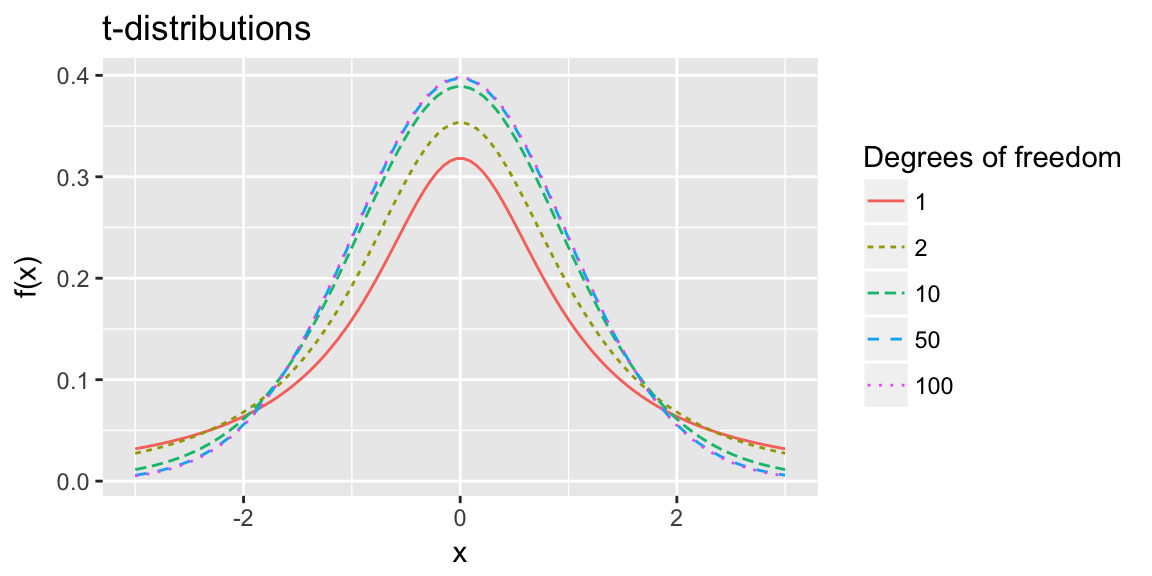# T-tests ST551 Lecture 10

## Warm up: from last times slides:

A random sample of $$n = 25$$ Corvallis residents had an average IQ score of 104. Assume a population variance of $$\sigma^2 = 225$$. What’s the mean IQ for Corvallis residents? Is it plausible the mean for Corvallis residents is greater than 100?

Find point estimate, Z-stat and p-value, and 95% confidence interval

qnorm(0.975) = 1.96
pnorm(1.33) = 0.9082409

# t-tests

## Inference for a population mean

What do we do if we don’t know $$\sigma^2$$? Realistically this is always the case

We can estimate $$\sigma^2$$, just like we estimated $$\mu$$:

• We used the sample mean to estimate the population mean
• We can use the sample variance to estimate the population variance

## Sample variance

The sample variance for a sample $$Y_1, \ldots, Y_n$$ is: $s^2 = \frac{1}{n-1}\sum_{i=1}^n \left(Y_i - \overline{Y}\right)^2$

Facts about the sampling distribution of the sample variance:

• The mean is $$\sigma^2$$, i.e. $$s^2$$ is an unbiased estimate of $$\sigma^2$$
• As the sample size $$n$$ gets larger, $$s^2$$ gets closer and closer to the true population variance $$\sigma^2$$, i.e. $$s^2$$ is consistent estimate of $$\sigma^2$$

## t-statistic

If we replace $$\sigma^2$$ with $$s^2$$ in the Z-statistic for testing $$H_0: \mu = \mu_0$$, we get a t-statistic:

$Z(\mu_0) = \frac{\overline{Y} - \mu_0}{\sqrt{\sigma^2/n}} \rightarrow t(\mu_0) = \frac{\overline{Y} - \mu_0}{\sqrt{s^2/n}}$

## Reference distribution

We compared the Z-statistic to N$$(0, 1)$$

• Why? N$$(0, 1)$$ is the distribution we expect for $$Z$$, when the null hypothesis is true

What should we compare a t-statistic to?

• $$s^2$$ will sometimes be smaller than $$\sigma^2$$, sometimes bigger
• Introduces additional variability into our test statistic

## t-distribution

The null distribution for a t-statistic is the t-distribution.

the t-distribution is a family of distributions defined by a single parameter the degrees of freedom

Notation:

• $$t_(1)$$ - t-distribution with 1 degree of freedom
• $$t_(3)$$ - t-distribution with 3 degrees of freedom
• $$t_(v)$$ - t-distribution with $$v$$ degree of freedom

Looks a lot like a Standard normal but with heavier tails and sharper peak.

## t-distributionAs $$v \rightarrow \infty$$ $$t_{(v)}$$ approaches the Standard Normal density.

## t-test: Inference for population mean

• If the population is exactly Normal:

• $$\overline{Y}$$ exactly Normal.
• t-statistic is exactly a t-distribution with $$n-1$$ degrees of freedom
• If population is anything with finite variance:

• $$\overline{Y}$$ approximately Normal,
• t-statistic approximately t-distribution with $$n-1$$ d.f.

## t-test: Inference for population mean

Rather than coming from a Standard Normal:

• Rejection region critical values come from t-distribution quantiles
• CI multipliers come from t-distribution quantiles
• P-values come from the cumulative distribution function of the t-distribution

In R: pt(q, df), qt(p, df), dt(x, df)

## t-test: Summary

Data Setting One sample, no explanatory variable $$Y_1, \ldots, Y_n$$ i.i.d from population with unknown variance $$\sigma^2$$

Null hypothesis $$H_0: \mu = \mu_0$$

Test statistic $t(\mu_0) = \frac{\overline{Y} - \mu_0}{\sqrt{s^2/n}}$

Reference distribution $$t(\mu_0) \dot \sim t_{(n-1)}$$

## t-test: Summary

Rejection Region for level $$\alpha$$ test

One sided $$H_A: \mu < \mu_0$$ Two sided $$H_A: \mu \ne \mu_0$$ One sided $$H_A: \mu > \mu_0$$
$$t(\mu_0) < t_{(n-1)\alpha}$$ $$|t(\mu_0)| > t_{(n-1)1-\alpha/2}$$ $$t(\mu_0) > t_{(n-1)1 - \alpha}$$

$$t_{(n-1) \alpha}$$ = qt(alpha, df = n - 1)

## t-test: Summary

p-values given an observed $$t(\mu_0) = t$$

One sided $$H_A: \mu < \mu_0$$ Two sided $$H_A: \mu \ne \mu_0$$ One sided $$H_A: \mu > \mu_0$$
$$F_t(t; n-1)$$ $$2\left(1 - F_t(|t|; n-1)\right)$$ $$1 - F_t(t; n-1)$$

$$F_t(t; n-1)$$ = pt(t, df = n-1)

Confidence Intervals $$(1-\alpha)100\%$$

$\left( \overline{Y} - t_{(n-1)1 - \alpha/2} \, \sqrt{\frac{s^2}{n}}, \, \overline{Y} + t_{(n-1)1 - \alpha/2} \, \sqrt{\frac{s^2}{n}} \right)$

## Standard error

$Var(\overline{Y}) = \frac{\sigma^2 }{n}$ We estimated $$\sigma^2$$ with $$s^2$$, hence estimate $$Var(\overline{Y})$$ with

$\widehat{Var}(\overline{Y}) = \frac{s^2}{n}$

Square root of this, often called, standard error of the mean:

$\text{SE}(\overline{Y}) = \frac{s}{\sqrt{n}}$

In general standard error refers to the estimated standard deviation of an estimator.

## Next time

Population proportions (a special case of population means)